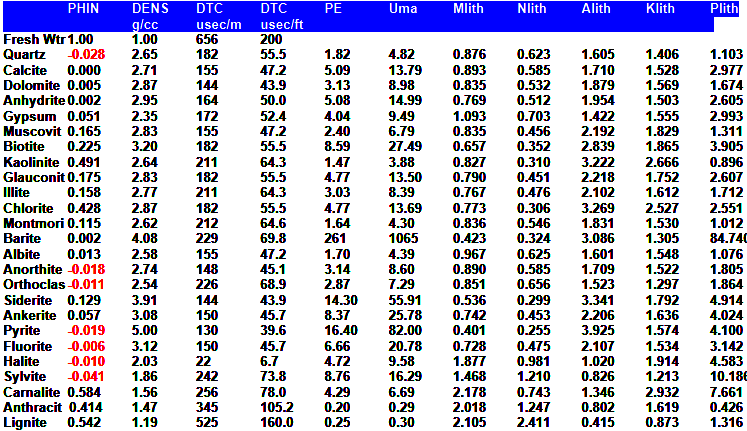Sonic Neutron Crossplot
The sonic neutron crossplot model  is used to estimate porosity when the density neutron crossplot method cannot be used, due to lack of density log or bad density data caused by rough hole conditions.. The sonic neutron crossplot method works well when shale volume, matrix rock properties, and sonic compaction effects  are accurately known. If both density and neutron logs are available, a superior model that does not require matrix rock properties is the Shale Corrected Density Neutron Complex Lithology Crossplot Method. The Meta/Kwik spreadsheet for this model is available at

The sonic neutron crossplot method involves the simultaneous solution of the sonic and neutron response equations for porosity. They are similar in form to the density neutron pair, and will not be repeated here.

Since both logs respond similarly to shale, the formulae do not have much accuracy in very shaly sands.

Gas effect is similar to the density neutron crossplot, so gas may be corrected for.Sonic Neutron CrossplOT POROSITY
Calculate compaction correction for sonic.
For Metric units (usec/m)
1A: KCP = max (1, DTCSH / 328
OR For English units (usec/ft)
1B: KCP = max (1, DTCSH / 100
Calculate sonic porosity.
2: PHIS = (DTC - DTCMA) / (DTCW - DTCMA) / KCP

Calculate shale corrected sonic porosity:
3: PHISSH = (DTCSH - DTCMA) / (DTCW - DTCMA) / KCP
4: PHIsc = PHIS - Vsh * PHISSH

Calculate shale corrected neutron porosity:
5: PHInc = PHIN - Vsh * PHINSH

Check for gas crossover using PHInc and PHIsc.
If no gas crossover, that is
6: IF PHInc >= PHIsc
7: THEN E = 0.5 - 10 ^ (-5 PHInc - 0.3)
8: AND G = -0.146
9: AND PHIxsn = (G * PHInc - E * PHIsc) / (G - E)

If gas crossover occurs, that is:
10: IF PHInc < PHIsc
11: THEN PHIxsn = ((PHIsc ^ 2 + PHInc ^ 2) / 2) ^ 0.5

Where:
DTCSH = sonic log shale value for compaction correction (usec/ft or usec/m)
KCP = compaction factor (fractional)
DTC = sonic log reading (usec/ft or usec/m)
DTCMA = travel time in rock matrix (usec/ft or usec/m)
DTCSH = sonic log reading in shale (usec/ft or usec/m)
DTCW = travel time in water (usec/ft or usec/m)
E = neutron pseudo matrix point
G = sonic pseudo matrix point
PHIN = neutron log reading (fractional)
PHINSH = neutron log reading in shale (fractional)
PHInc = porosity from neutron log corrected for shale (fractional)
PHIS = porosity from sonic log (fractional)
PHIsc = porosity from sonic corrected for shale (fractional)
PHISSH = sonic porosity in shale (fractional)
PHIxsn = porosity from sonic neutron crossplot (fractional)
Vsh = shale volume (fractional)COMMENTS:
The overall layout of the neutron sonic crossplot is shown below.Chart for Sonic Neutron Porosity Model - shale corrected data must be entered

The gas correction represents a 45 degree line (in porosity units) on the crossplot. The method is best used in carbonates with or without gas, and is inappropriate for shaly sand.

Thismethod is especially useful in cased holes where a shear sonic and neutron log can be run through casing.NUMERICAL EXAMPLE:
1. Assume data for Sand "D".
DTC = 300 usec/m
PHIN = 0.28
KCP = 1.00
DTCSH = 328 usec/m
PHINSH = 0.30
Vsh = 0.33
DTCMA = 182 usec/m
DTCW = 616 usec/m
no matrix offset
PHIS = (300 - 182) / (616 - 182) / 1.0 = 0.27
PHISSH = (328 - 182) / (616 - 182) / 1.0 = 0.33
PHIsc = 0.27 - 0.33 * 0.33 = 0.16
PHInc = 0.28 - 0.33 * 0.30 = 0.18
E = -0.5 - 10 ^ (0-5 * 0.18 - 0.3) = 0.43
G = -0.146
PHIxsn = (-0.146 * 0.18 - 0.43 * 0.16) / (-0.146 - 0.54) = 0.165MATRIX ROCK PROPERTIESPage Views ---- Since 01 Jan 2015
Copyright 2023 by Accessible Petrophysics Ltd.
CPH Logo, "CPH", "CPH Gold Member", "CPH Platinum Member", "Crain's Rules", "Meta/Log", "Computer-Ready-Math", "Petro/Fusion Scripts" are Trademarks of the Author